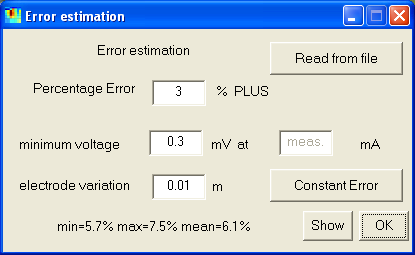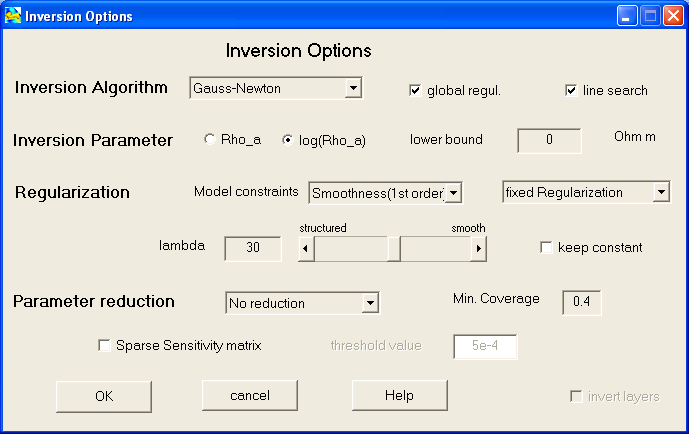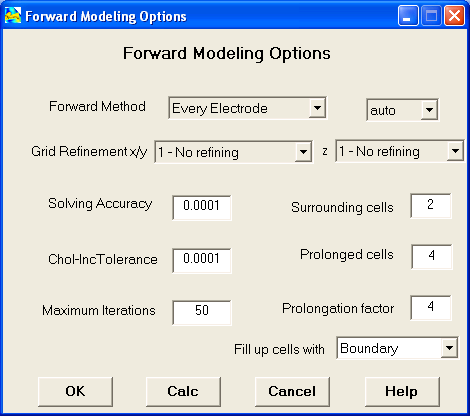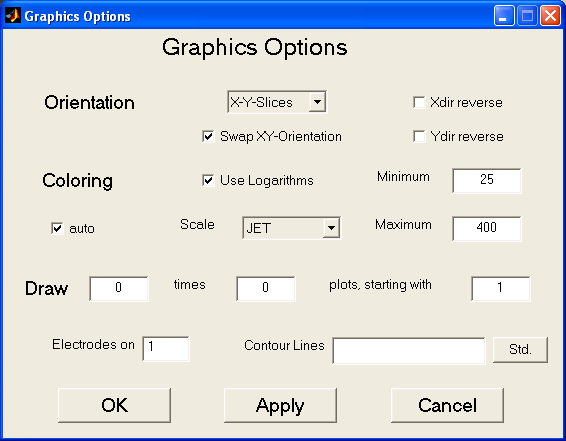Start Page Menu Items Interactive Features Hotkeys Tutorial

### Set Error

 Knowing the accuracy of the data points can significantly increase the interpretation of geophysical data. If errors (in terms of standard deviations) are not measured, it is possible to estimate. The (relative) data erros are supposed to consist of two parts, one percentage error plus an voltage error considering the limited accuracy of the field equipment. The voltage error is divided by the driving current and multiplied with the configuration factor giving large errors for high k-values (such as dipole-dipole) and small errors for low k-values (such as wenner). If no errors are given in the data file, an error estimation dialog is called. It is also possible to assume equal errors for the data or to read the standard deviation from text file.### Data Reweighting

The occurence of individual data with bad quality can severly limit the data fit in an least squares inversion based on minimization of the misfits L2-squared norm. The use of the L1-norm is much less sensitive to outliers and therefore denoted with the term robust inversion. However L1-inversion is much more difficult to implement. As an alternative the data weighting can be changed during inversion to simulate robust methods, generally known as iteratively reweighting. Her the ratio of L1 and L2 misfit is used (manually) to downweight error of data which could not be explained in the inversion process. It is recommended to use it in case of a heterogeneous misfit distribution after a certain number of iterations and to continue inversion process.

### Inversion Options

In the inversion options dialog defines the setup for treating the inverse problem.Inversion Algorithm

1. Several inversion algorithms are provided
• Gauss-Newton inversion is the standard algorithm
• Truncated SVD is based on singular value decomposition (only for very small datasets!)
• SIRT (simultaneous iterative reconstruction technique)
• Truncated least squares (alternative to Gauss-Newton without explicit constraints)
2. use a global regularization technique (recommended) instead of linearized problems
3. use an inexact line search procedure to improve convergence

Inversion Parameter

1. one can choose between logarithmic (default) and linear parameters
2. in case of logarithmized parameters a lower bound can be defined to prevent the resistivities from undergoing a certain value (must be greater than smallest apparent resistivity!)

Regularization

1. The type of model constraints are defined: mimimum length, coverage weighted parameters and different smoothness constraints are used to restrict the model characteristics
2. Here is defined how the regularization strength is defined. Possible is
• A fixed regularization strength is determined by the slider value below.
• An optimal value is chosen via the L-curve criterion.
• The user can choose the value interactively while watching the corresponding models.
For the last two methods the lambda value below defines the minimum value. Note tha these methods need more time, because solutions for a series of lambda have to be obtained.
3. The most important option is the regularization strength. It can be varied by moving the slider or typing in the value directly. Usual values are in the range of 10-30, sometimes less (1-3). Bad data or error estimates may require values of a few 100.
4. With the "keep constant" button the chosen (manual or automatic) value is fixed after the first iteration.

Parameter Reduction

A reduction of the number of free parameters can decrease computation time and improve the results. This can be done by deleting bad covered data (under minimum coverage) or by combining cells in greater depths (default) or by both methods.
• Generally the Jacobian matrix containg the sensitivities has full density and needs lots of memory. The memory can be significantly reduced by neglecting small values under the given threshold value. It is recommended for large data sets. However the choice of the threshold value (1e-3 to 1e-4) is rather experimental.

### Forward Options

The forward calculation is carried out using finite differences (Dey&Morrison, 1979). By the singulariy removal technique (Lowry, 1989) the computational effort is reduced.1. Generally, the potentials for every electrode are computed and superposed for the output. It is also possible (but slower) to simulate real dipoles.
As an approximation (which helds for small contrasts) the forward calculation can also be done using the sensitivity matrix as linearized forward operator.
2. The resulting (and reordered) systems of equations may be solved by conjugate gradients or by direct methods. The latter are very fast for many sources but need lots of memory. The auto button chooses the method depending on the system size.
3. When the model contains high resistivity contrasts, the solution can result in bad accuracy. This can be avoided by grid refinement, both of the z-axis (manually) and the x-axis (manually or automatically such that equal-sized blocks are created)
4. In case of conjugate gradient some parameters are defined
• Solution accuracy is the relative residual. 1e-4 is enough for practical purposes.
• The quality of the uncomplete Cholesky preconditioner. Small values save memory but needs more memory, too small values may need much memory. The optimum value is often close to 1e-4.
• The maximum iteration number limits an errorneous computation but is used seldom.
5. In every FD simulation it is necessary to enlarge the model to get a good approximation of boundary conditions. Is is done by several equal-sized "surrounding" cells and several "boundary" cells enlarged with a prolongation factor.
6. The boundary cells can be either filled with an exist layered model or the resistivity of the neighboring cells.

### Graphics Options

This dialog defines, how the model and data sections are plotted.Coloring can be done automatical or manually by giving minimum and maximum value. Several color maps can be chosen. The user can decide, whether to draw electrodes or not. The x-axis may be reversed. As default option the logarithms of resistivity are plotted.
The default way to plot model cells is with colored boxes (because it shows how the model really looks like), but filled contour lines or smoothed image are also possible.

### Save Options

All Options can be saved as default values.

### Reset Options

All options are reset to the default (by user saved) options.